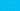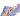PricingResources

# Guide to the compound interest formula

Written by

If you’re looking for financial products with the most attractive interest rates, the compound interest formula is an important equation to know. Keep reading to learn more about this formula and how it’s applied.

## What is compound interest?

Compound interest is additional interest earned on your account’s existing interest rates – interest on interest. By contrast, simple interest refers to interest on principal funds deposited into your account. In adding this extra layer of interest, compounding allows for faster growth than you would see with simple interest alone. You not only earn interest on your principal, but also on the principal’s earned interest.

## How to calculate compound interest

To calculate the rate at which your compound interest will accrue, you need to factor in several components. This includes the frequency of compounding, the interest rate, and the initial amount of money deposited (principal). The more compounding periods you have, the higher your compound interest will be.

The compound interest formula works by multiplying your initial principal by one plus the annual interest rate, raised to the number of compound periods. You’ll then need to subtract the initial loan amount from this value.

## Compound interest equation

Figuring out how to calculate compound interest is easier when you can see it laid out in an equation. Here’s the compound interest formula for quick calculations:

• A = final amount

• P = principal balance

• R = interest rate (as decimal)

• N = number of times interest will be applied per time period

• T = number of time periods elapsed

As an example, imagine that you have deposited a principal amount of \$10,000 with an annual interest rate of 5%, which will be compounded monthly over a period of five years. You can then plug this into the compound interest equation to calculate the interest you would earn:

You’d earn \$2,833.59 on top of your initial investment amount through compound interest.

If you were to track the compound interest formula in a graphic form, you’d see the significant difference between compound and simple interest. While simple interest would show the same interest amount accumulating over time, compound interest starts to grow exponentially.

It can be tricky to work out the compound interest equation by hand, but fortunately there are numerous online calculators to take care of the math side for you. Another option is to use Excel to calculate compound interest.

## The importance of compounding frequency

The number of compounding periods makes a big difference in your resulting sum. Interest can be compounded according to any schedule of frequency, whether it’s daily, weekly, monthly, or annually. For the typical savings account, a daily schedule is usually followed, while business loans and credit card accounts often use a monthly schedule.

Over the long term, compound interest can give your investment returns a significant boost. Look for a more frequent compounding schedule to maximize returns. On the other hand, if you’re borrowing money, you’d want to choose a financial product with less frequent compounding schedules.

Continuously compounding interest, as the name suggests, adds interest to your principal on an ongoing basis. In reality, interest that’s compounded continuously doesn’t add up to much more than a daily frequency. However, it can be worthwhile if you want to earn money in a single day.

## The bottom line

Compound interest is most beneficial for investors, who can receive higher returns on bank accounts and bonds. Whether your interest is compounded continuously or annually, knowing the ins and outs of the compound interest formula can help you gain greater understanding of how your principal grows.

## We can help

GoCardless helps you automate payment collection, cutting down on the amount of admin your team needs to deal with when chasing invoices. Find out how GoCardless can help you with ad hoc payments or recurring payments.## Interested in automating the way you get paid? GoCardless can help

Contact sales

Sales

Contact Sales

+1(415) 523-2279

Support

help@gocardless.com

+1 (628) 241-0044